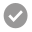Papers Repository of the International Centre for Numerical Methods in Engineering (CIMNE)

##A residual correction method based on finite calculus

Abstract

In this paper, a residual correction method based upon an extension of the finite calculus concept is presented. The method is described and applied to the solution of a scalar convection‐diffusion [...]

•10
Papers Repository of the International Centre for Numerical Methods in Engineering (CIMNE)

##A finite point method for elasticity problems

Abstract

The basis of the finite point method (FPM) for the fully meshless solution of elasticity problems in structural mechanics[...]
•23
Papers Repository of the International Centre for Numerical Methods in Engineering (CIMNE)

##Stress, strain and energy splittings for anisotropic elastic solids under volumetric constraints

Abstract

We define stress and strain splittings appropriate to linearly elastic anisotropic materials with volumetric constraints. The treatment includes rigidtropic materials, which [...]

•7
Papers Repository of the International Centre for Numerical Methods in Engineering (CIMNE)

##An analytical–numerical approach to simulate the dynamic behaviour of arbitrarily laminated composite plates

Abstract

A general analytical–numerical approach developed for the dynamical analysis of unsymmetrically laminated plates of general quadrilateral planforms is presented in this work. An arbitrary [...]

•0
Papers Repository of the International Centre for Numerical Methods in Engineering (CIMNE)

##On the geometric character of stress in continuum mechanics

Abstract

This paper shows that the stress field in the classical theory of continuum mechanics may be taken to be a covector-valued differential two-form. The balance laws and other fundamental laws of continuum mechanics may be neatly rewritten in terms of this geometric stress. A geometrically [...]

•2
Papers Repository of the International Centre for Numerical Methods in Engineering (CIMNE)

##A superconvergent hybridisable discontinuous Galerkin method for linear elasticity

Abstract

The first superconvergent hybridisable discontinuous Galerkin method for linear elastic problems capable of using the same degree of approximation [...]

•0
Eugenio Oñate's Journal Papers

##Accurate modelling of the elastic behavior a continuum with the Discrete Element Method

Abstract

The Discrete Element Method (DEM) has been used for modeling continua, like concrete or rocks. However, it requires a big calibration effort, even to capture just the linear elastic behavior of a continuum modelled via the classical force-displacement relationships at the contact [...]

•74
•5/5
Papers Repository of the International Centre for Numerical Methods in Engineering (CIMNE)

##An accurate nonlocal bonded discrete element method for non linear analysis of solids. Application to concrete fracture tests (preprint)

Abstract

We present a numerical procedure for elastic and non linear analysis (including fracture situations) of solid materials and structures using the Discrete Element Method (DEM). It can be applied to strongly cohesive frictional materials such as concrete and rocks. The method consists [...]

•42
Revista Internacional de Métodos Numéricos para Cálculo y Diseño en Ingeniería

##Finite volume for stress analysis upon polygonal, unstructured and non conforming meshes

Abstract

Within this paper we discuss a numerical strategy to solve the elasticity problem upon unstructured and non conforming meshes, allowing all kinds of flat-faced elements (polygons in 2D and polyhedra in 3D). The core of the formulation relies on two numerical procedures 1) the Control [...]

•172
•5/5
Papers Repository of the International Centre for Numerical Methods in Engineering (CIMNE)

##Enhanced balancing Neumann-Neumann preconditioning in computational fluid and solid mechanics

Abstract

In this work, we propose an enhanced implementation of balancing Neumann-Neumann (BNN) preconditioning together with a detailed numerical comparison against the balancing domain decomposition by constraints (BDDC) preconditioner. As model problems, we consider the Poisson and linear [...]

•3